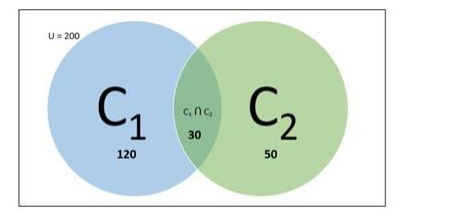# There are 200 individuals with a skin disorder,

Question:

There are 200 individuals with a skin disorder, 120 had been exposed to the chemical C1, 50 to chemical C2, and 30 to both the chemicals C1 and C2. Find the number of individuals exposed to

(i) Chemical $\mathrm{C}_{1}$ but not chemical $\mathrm{C}_{2}$

(ii) Chemical $\mathrm{C}_{2}$ but not chemical $\mathrm{C}_{1}$

(iii) Chemical $C_{1}$ or chemical $C_{2}$

Solution:

Given:

Total number of individuals with skin disorder $=200$

Individuals exposed to chemical $C_{1}=120$

Individuals exposed to chemical $C_{2}=50$

Individuals exposed to chemicals $C_{1}$ and $C_{2}$ both $=30$

To Find:

(i) Individuals exposed to Chemical $C_{1}$ but not $C_{2}$

Let us consider,

Total number of individuals with skin disorder = n(C) = 200

Individuals exposed to chemical $C_{1}=n\left(C_{1}\right)=120$

Individuals exposed to chemical $\mathrm{C}_{2}=\mathrm{n}\left(\mathrm{C}_{2}\right)=50$

Individuals exposed to chemicals $C_{1}$ and $C_{2}$ both $=n\left(C_{1} \cap C_{2}\right)=30$

Individuals exposed to Chemical $C_{1}$ but not $C_{2}=n\left(C_{1}-C_{2}\right)$

Venn diagram:Now

$n\left(C_{1}-C_{2}\right)=n\left(C_{1}\right)-n\left(C_{1} \cap C_{2}\right)$

$=120-30$

$=90$

Therefore, number of individuals exposed to chemical $C_{1}$ but not $C_{2}=90$

(ii) Individuals exposed to Chemical $C_{2}$ but not $C_{1}$

Let us consider number of Individuals exposed to Chemical $C_{2}$ but not $C_{1}=n\left(C_{2}-C_{1}\right)$

Now,

$\mathrm{n}\left(\mathrm{C}_{2}-\mathrm{C}_{1}\right)=\mathrm{n}\left(\mathrm{C}_{2}\right)-\mathrm{n}\left(\mathrm{C}_{1} \cap \mathrm{C}_{2}\right)$

$=50-30$

$=20$

Therefore, number of individuals exposed to chemical $C_{2}$ but not $C_{1}=20$

(iii) Individuals exposed to Chemical $C_{1}$ or chemical $C_{2}$

Let us consider number of Individuals exposed to Chemical $C_{1}$ or chemical $C_{2}=$ $n\left(C_{1} \cup C_{2}\right)$

Now,

$n\left(C_{1} \cup C_{2}\right)=n\left(C_{1}\right)+n\left(C_{2}\right)-n\left(C_{1} \cap C_{2}\right)$

$=120+50-30$

$=140$

Therefore, number of individuals exposed to chemical $\mathrm{C}_{1}$ or $\mathrm{C}_{2}=140$